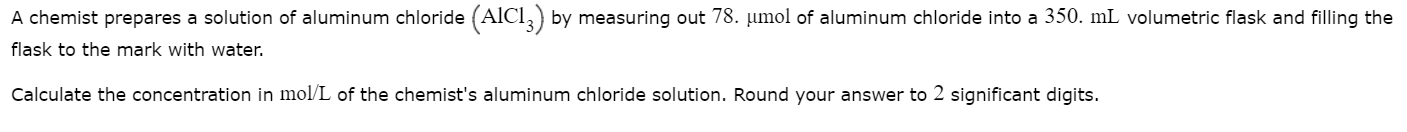# A chemist prepares a solution of aluminum chloride (AICI,) by measuring out 78. umol of aluminum chloride into a 350. mL volumetric flask and filling theflask to the mark with water.Calculate the concentration in mol/L of the chemist's aluminum chloride solution. Round your answer to 2 significant digits.

Question
14 views

photo attachedhelp_outlineImage TranscriptioncloseA chemist prepares a solution of aluminum chloride (AICI,) by measuring out 78. umol of aluminum chloride into a 350. mL volumetric flask and filling the flask to the mark with water. Calculate the concentration in mol/L of the chemist's aluminum chloride solution. Round your answer to 2 significant digits. fullscreen
check_circle

Step 1

The molarity or the concentration can be defined as the ratio of number of moles of solute which are present per liter of the given solution. The SI units of molarity are moles/L. The mathematical formula as follows:

Step 2

In the given problem, the number of moles of AlCl3 which have been dissolved by the chemist is given to be 78 micromole.Now,

Step 3

The volume of the solution is given to be 350 mL=0.35 L.

The mo...

### Want to see the full answer?

See Solution

#### Want to see this answer and more?

Solutions are written by subject experts who are available 24/7. Questions are typically answered within 1 hour.*

See Solution
*Response times may vary by subject and question.
Tagged in

### Physical Chemistry